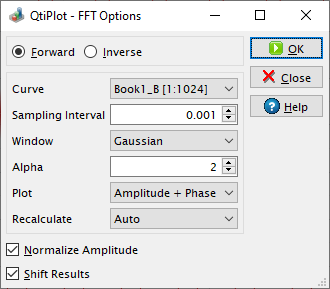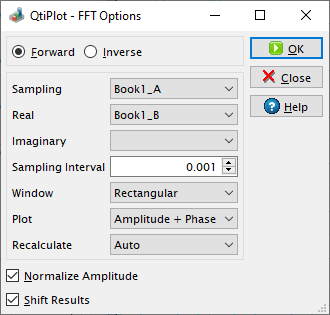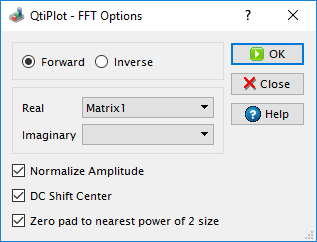# Fast Fourier Transform Dialog

The FFT... dialog box can be used on a plot, on a table or on a matrix. It is used to compute either the direct or inverse FFT. See the FFT section in the Analysis chapter for an example.

Figure 5-74. The FFT... dialog box for a curve.QtiPlot will create a new plot window containing the FFT amplitude curve, along with a new table which contains the real part, the imaginary part, the amplitude, and the phase of the FFT.

If the Normalize Amplitude check box is on, the amplitude curve is normalized to 1. If the Shift Results check box is on, the frequencies are shifted in order to obtain a centered x-scale.

Figure 5-75. The FFT... dialog box for a table.When performing an FFT on a table, you must select the sampling column (X-values) and one (for simple real values) or two (for complex values) columns of Y-values. Complex numbers have the real part of the Y-values in the first column and the imaginary part of the Y-values in the second column. If Y-values are simple reals, you don't have to select a column for the imaginary part. You can leave the combo box for the second column empty in this case.

By default, the Sampling Interval corresponds to the interval between X-values. Giving a smaller value makes no sense, but you can increase this value in order to sample fewer values.

On matrices QtiPlot performs a two dimensional FFT using two sampling intervals: one that corresponds to the interval between the X-values and a second one corresponding to the interval between the Y-values. For more details about the two dimensional FFT please read the following article by Paul Bourke: http://paulbourke.net/miscellaneous/dft/.

Figure 5-76. The FFT... dialog box for a matrix.If the dimensions of the input matrix are not powers of 2, you have the option to resize it for a more efficient FFT, by checking the box Zero-pad to nearest power of 2 size. The new dimensions are calculated to be the closest powers of 2 from the initial dimensions of the matrix and the extra data values used for the FFT are set to zero.

As the result of the FFT operation on a matrix QtiPlot creates a new matrix window containing the amplitude of the FFT, along with two other matrices which contain the real and the imaginary parts of the FFT.